## Monday, March 16, 2009

### MSF CSP: Tiling Squares

The tiling problem is not very amenable for solving by MIP solvers (see tiling.pdf). In this problem we try to cover an (n × n) square with smaller squares. In a MIP we need big-M formulations to express the non-overlap condition. With CSP we can write this down more directly and conveniently. Instead of using something like: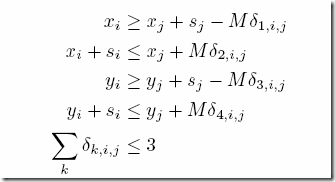we can write using Microsoft Solver Foundation:

Foreach[{i,T},{j,i+1,T},
x[i]>=x[j]+Side[j] |
x[i]+Side[i]<=x[j] |
y[i]>=y[j]+Side[j] |
y[i]+Side[i]<=y[j]
]

Here the vertical bar means OR. A small (7 × 7) problem can be implemented quickly as follows: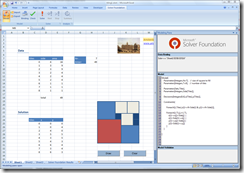Here we already used knowledge about which tiles to use. We only needed to determine where to place them. The real problem is slightly more complicated: we don’t know which tiles to use in advance. Below, we are looking for a configuration for the (9 x 9) problem where no tile can be used more than three times. This is number h(9) in Integer Square Tilings and a(9) in sequence A036444. To formulate this problem we add boolean variables b(i) indicating if tile i is being used.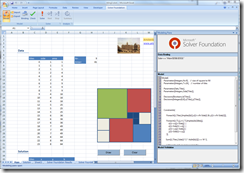Also we added an extra constraint to reduce symmetry (this caused significant speed-up). The solution times with and without the symmetry breaking constraint are: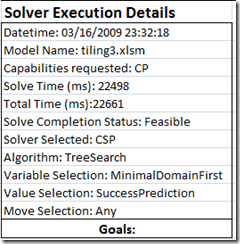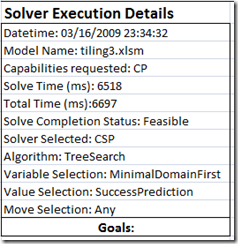Without symmetry breaking constraint With symmetry breaking constraint

I did not make an exhaustive examination of all solver options, so there may be better performance possible. The complete model looks like:

``Model[    Parameters[Integers,N=9],   // size of square to fill    Parameters[Integers,T=24],   // number of tiles      Parameters[Sets,Tiles],       Parameters[Integers,Side[Tiles]],           Decisions[Booleans,b[Tiles]],    Decisions[Integers[0,8],x[Tiles],y[Tiles]],       Constraints[           Foreach[{i,Tiles},Implies[b[i],x[i]<=N-Side[i] & y[i]<=N-Side[i]]],           // Note: Use T instead of Tiles         Foreach[{i,T},{j,i+1,T},Implies[b[i]&b[j],             x[i]>=x[j]+Side[j] |             x[i]+Side[i]<=x[j] |             y[i]>=y[j]+Side[j] |             y[i]+Side[i]<=y[j]         ]],          Sum[{i,Tiles},Side[i]^2 * AsInt[b[i]]] == N^2,                  // this is to reduce some symmetry        Foreach[{i,T},{j,i+1,T},Implies[b[i]&b[j]&(Side[i]==Side[j]),x[j]>=x[i]]]    ]  ]``

We use the Implies[] construct here to implement if-then. Note that we used T instead of Tiles when we needed to operate on the upper-triangle of the (i,j) pairs. In this case we could not use Tiles as OML then assumes the indices are non-numeric (well, they are actually integers).

Update: more symmetry breaking constraints and their effect on solution times are discussed in this follow-up.